Include Top

# How do I create a One Sample Wilcoxon Signed Rank Test - Exact in SigmaXL?

This tool is used to calculate exact P-Values. Typically this would not be necessary unless the sample sizes were smaller (N <= 15 for Wilcoxon), but this gives continuity on the example.

1. Open Customer Data.xlsx, select Sheet 1 tab (or press F4 to activate last worksheet). Click SigmaXL > Statistical Tools > Nonparametric Tests – Exact > 1 Sample Wilcoxon - Exact. If necessary, check Use Entire Data Table, click Next.
2. Ensure that Stacked Column Format is selected. Select Overall Satisfaction, click Numeric Data Variable (Y) >>; select Customer Type, click Optional Group Category (X) >>.
3. Enter 3.5 for the Null Hypothesis H0: Median value. Set Ha as Not Equal To.

4.5. Click OK. Results:

6.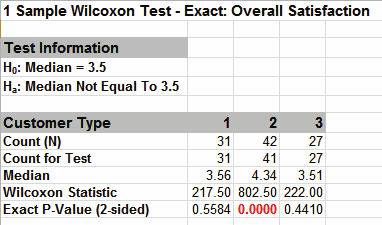The Wilcoxon Statistics are identical to the above “large sample” or “asymptotic” results. The Exact P-Values are close but slightly different. This was expected because the sample sizes are reasonable (N > 15), so the “large sample” P-Values are valid using a normal approximation for the Wilcoxon Statistic.

Note, if Count (N) is greater than 1000, the Exact P-Value is estimated using a continuity -corrected normal approximation. Since the Wilcoxon Exact P-Value is computed very quickly for sample sizes as large as 1000, Monte Carlo P-Values are not required.

7. Now we will consider a small sample problem. Open Nonnormal Task Time Difference – Small Sample.xlsx. A study was performed to determine the effectiveness of training to reduce the time required to complete a short but repetitive process task. Fifteen operators were randomly selected and the difference in task time was recorded in seconds (after training – before training). A negative value denotes that the operator completed the task in less time after training than before.
8. Click SigmaXL > Graphical Tools > Histograms & Descriptive Statistics. If necessary, check Use Entire Data Table. Click Next. Select Difference (Seconds), click Numeric Data Variables (Y) >>. Click OK.This small sample data fails the Anderson Darling Normality Test (P-Value = .023). Note that this is due to the data being uniform or possibly bimodal, not due to a skewed distribution. Now we will perform a 1 Sample t-Test and review the assumptions.

9. Select Task Time Difference tab (or press F4 to activate last worksheet). Click SigmaXL > Statistical Tools > 1 Sample t-Test & Confidence Intervals. If necessary, check Use Entire Data Table, click Next.
10. Ensure that Stacked Column Format is selected. Select Difference (Seconds), click Numeric Data Variable (Y) >>.
11. This is a one sided test because we have no reason to expect that the task time will increase, so the Null Hypothesis H0: μ = 0; and the Alternative Hypothesis Ha: μ < 0. Enter 0 for the Null Hypothesis H0: Mean value. Select Ha as Less Than, Confidence Level = 95.0%, and check Display Test Assumptions Report.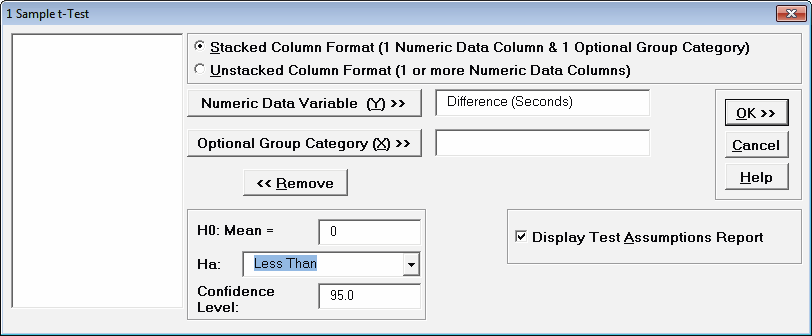12. Click OK.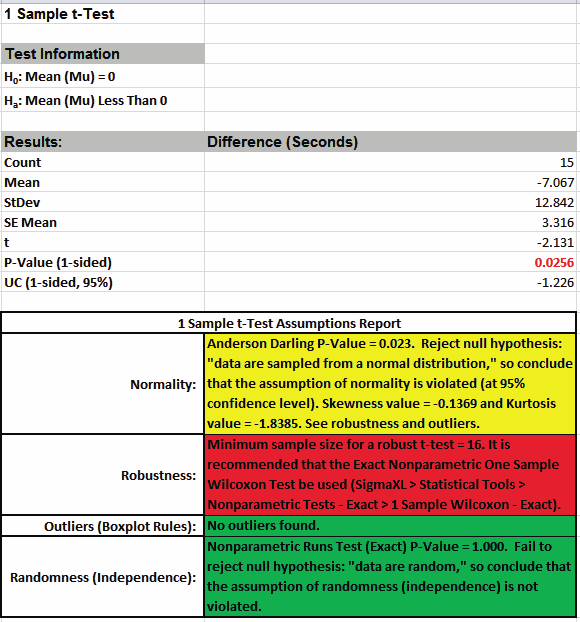The 1 Sample t-Test Assumptions Report highlights that the data are not normal, but note that Kurtosis equal to -1.84 is the issue here, not Skewness. This was observed in the Histogram above with the data being uniform or possibly bimodal.

The sample size is too small for a robust t-Test, so the Exact One Sample Wilcoxon Test is recommended. The Wilcoxon Test is recommended over the Sign Test because it is a more powerful test and meets the requirement that the data be symmetrical. The Exact test is recommended because the sample size is very small (N <= 15).

13. Select Task Time Difference tab (or press F4 to activate last worksheet). Click SigmaXL > Statistical Tools > Nonparametric Tests – Exact > 1 Sample Wilcoxon - Exact. If necessary, check Use Entire Data Table, click Next.
14. Ensure that Stacked Column Format is selected. Select Difference (Seconds), click Numeric Data Variable (Y) >>. Enter 0 for the Null Hypothesis H0:Median value. Select Ha as Less Than.
15. Click OK. Results: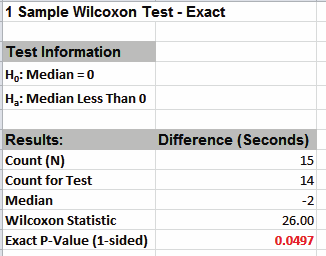With the P-Value = .0497 we reject H0 and conclude that the Median Task Time Difference is significantly less than 0, so the training is effective.By way of comparison we will now rerun the analysis using the large sample (asymptotic) Wilcoxon test.

16. Select Task Time Difference tab (or press F4 to activate last worksheet). Click SigmaXL > Statistical Tools > Nonparametric Tests > 1 Sample Wilcoxon. If necessary, check Use Entire Data Table, click Next.
17. Ensure that Stacked Column Format is selected. Select Difference (Seconds), click Numeric Data Variable (Y) >>. Enter 0 for the Null Hypothesis H0:Median value. Select Ha as Less Than.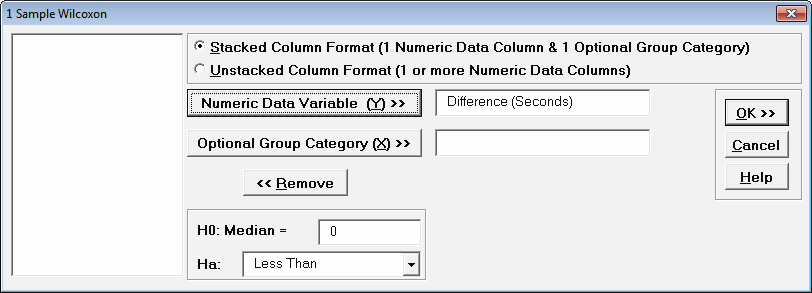18. Click OK. Results:Now with the P-Value = .0513 we incorrectly fail to reject H0.

The difference between exact and large sample P-Value is small but it was enough to lead us to falsely conclude that the training is ineffective.

In conclusion, whenever you have a small sample size and are performing a Nonparametric test, always use the Exact option.

# Web Demos

Our CTO and Co-Founder, John Noguera, regularly hosts free Web Demos featuring SigmaXL and DiscoverSim